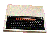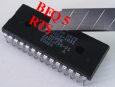×   Main Menu ALL The 8BS News Manuals (New menu) Links Worth a Look Tools Disc and Basic Webring Site Map 8BS Guestbook Old Guest Book Me The Barnsley Rovers# The BBC and Master Computer Public Domain Library

### A89C ACS

Submitted by Steve Fewell

Description:

Call routine &A8A1 to get the Float value (at the BASIC text pointer location, converting Integer to Float - if
the value was Integer) and calculate the Arc-Sine (ASN) result of the Float value.
Call routine &A8E1 to: set the FWA = (1.57079633 (constant at &BF2E) - FWA) and exit with A=#&FF (as the
result is a Floating-point value in the FWA).

The ACS calculation is simply:
ACS(x) = 1.57079633 - ASN(x)

Example 1: ACS(0)
FWA = ASN(FWA) = 0.0
FWA = 1.57079633 - FWA = 1.57079633

Example 2: ACS(0.25)
FWA = ASN(FWA) = 0.2526802
FWA = 1.57079633 - FWA = 1.3181161

Example 3: ASN(-0.75)
FWA = ASN(FWA) = -0.848062
FWA = 1.57079633 - FWA = 2.4188583

This diagram shows the relationship between the trig functions.

### Disassembly for the ACS routine

 A89C 032 161 168 20 A1 A8 JSR &A8A1 Get Float value and calculate ASN result A89F @ 128 064 80 40 BRA 64 --> &A8E1 FWA = 1.57079633 (constant at &BF2E) - FWA & exit with A=#&FFOr